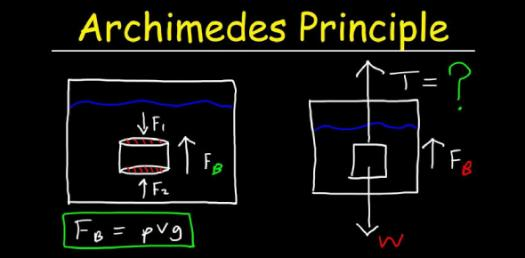# What Do You Know About Archimedes’ Principle And Buoyancy? Trivia Quiz

6 Questions | Total Attempts: 443SettingsCreate your own QuizWhat do you know about Archimedes’ principle and buoyancy? The principle states that the amount of fluid displaced when an object is placed in a fluid is equal to its weight. Do take up the quiz below and get to see if you know about the principle from your classes. Be sure to give each question the same amount of time.

• 1.
The weight of a stone in air s 0.95N. When it is completely submerged in water, its weight is 0.48N. What is the buoyant force acting on the rock when it is completely submerged in the water?
• A.

1.43 N

• B.

0.47 N

• C.

-1.43 N

• D.

-0.47 N

• 2.
Which are the following is FALSE about Archimedes' Principle?
• A.

A body at rest in a fluid is acted upon by a force pushing upward called the buoyant force, which is equal to the weight of the fluid that the body displaces.

• B.

If the body is completely submerged, the volume of fluid displaced is equal to the volume of the body.

• C.

If the body is only partially submerged, the volume of the fluid displaced is equal to the volume of the part of the body that is submerged.

• D.

The buoyant force is not equal to the weight of the fluid displaced and the buoyant force cannot be calculated.

• 3.
Name a force that acting on K
• A.

Electromagnetic Force

• B.

Buoyant Force

• C.

Nuclear Force

• D.

No forces acting on K

• 4.
These are the applications of Archimedes' Principle EXCEPT
• A.

Thermometer

• B.

Hydrometer

• C.

Submarine

• D.

Hot-air Ballon

• 5.
Why do you think an iceberg can float on the sea?
• A.

Weight of the iceberg > the weight of sea water displaced

• B.

Weight of the iceberg < the weight of sea water displaced

• C.

Weight of the iceberg = the weight of the sea water displaced

• D.

Weight of the iceberg = the weight of the air surrounding the iceberg

• 6.
What is the buoyant force on a block of copper with a volume of 0.0456m3 submerged in a tank of water? Given that the density of water is 1.0 x 103 kg/m3 and g = 10.0 m/s2
• A.

546 N

• B.

456 N

• C.

645 N

• D.

Question is not relevant

Related TopicsBack to top
×

Wait!
Here's an interesting quiz for you.